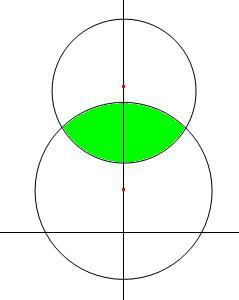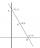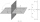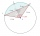# Circle

Circle is given by centre on S[-7; 10] and maximum chord 13 long.

How many intersect points have circle with the coordinate axes?

n =  0

### Step-by-step explanation:Did you find an error or inaccuracy? Feel free to write us. Thank you!Tips to related online calculators
Line slope calculator is helpful for basic calculations in analytic geometry. The coordinates of two points in the plane calculate slope, normal and parametric line equation(s), slope, directional angle, direction vector, the length of the segment, intersections of the coordinate axes, etc.
Looking for help with calculating roots of a quadratic equation?

#### You need to know the following knowledge to solve this word math problem:

We encourage you to watch this tutorial video on this math problem:

## Related math problems and questions:

• Coordinate axesDetermine the area of the triangle given by line -7x+7y+63=0 and coordinate axes x and y.
• XY triangleDetermine the area of a triangle given by line 7x+8y-69=0 and coordinate axes x and y.
• Points on circleIn the Cartesian coordinate system with the origin O is a sketched circle k /O; r=2 cm/. Write all the points that lie on a circle k and whose coordinates are integers. Write all the points that lie on the circle I / O; r=5 cm / and whose coordinates are
• Three pointsThree points A (-3;-5) B (9;-10) and C (2;k) . AB=AC What is value of k?
• Points in spaceThere are n points, of which no three lie on one line and no four lies on one plane. How many planes can be guided by these points? How many planes are there if there are five times more than the given points?
• Common chordTwo circles with radius 17 cm and 20 cm are intersect at two points. Its common chord is long 27 cm. What is the distance of the centers of these circles?
• Chord ABWhat is the chord AB's length if its distance from the center S of the circle k(S, 92 cm) is 10 cm?
• TestingStudents of high school have 10 points for each good solved task. The wrong answer is deducted by 5 points. After solving 20 tasks, student Michael had 80 points. How many tasks did he solve correctly and how many wrong?
• Chord BCA circle k has the center at the point S = [0; 0]. Point A = [40; 30] lies on the circle k. How long is the chord BC if the center P of this chord has the coordinates: [- 14; 0]?
• Equation with absSolve this equation with absolute value member: |3(3x-1)|=8
• Concentric circles and chordIn a circle with a diameter d = 10 cm, a chord with a length of 6 cm is constructed. What radius have the concentric circle while touch this chord?
• TangentsTo circle with a radius of 41 cm from the point R guided two tangents. The distance of both points of contact is 16 cm. Calculate the distance from point R and circle centre.
• SquarePoints A[9,9] and B[-4,1] are adjacent vertices of the square ABCD. Calculate the area of the square ABCD.
• Polygon - area coordinatesFind the perimeter and the area of the polygon with the given vertices. T (2,7), U (2,9), V (5,9), W (5,7)
• On a lineOn a line p : 3 x - 4 y - 3 = 0, determine the point C equidistant from points A[4, 4] and B[7, 1].
• LinesIn how many points will intersect 14 different lines, where no two are parallel?
• 3d vector componentThe vector u = (3.9, u3) and the length of the vector u is 12. What is is u3?